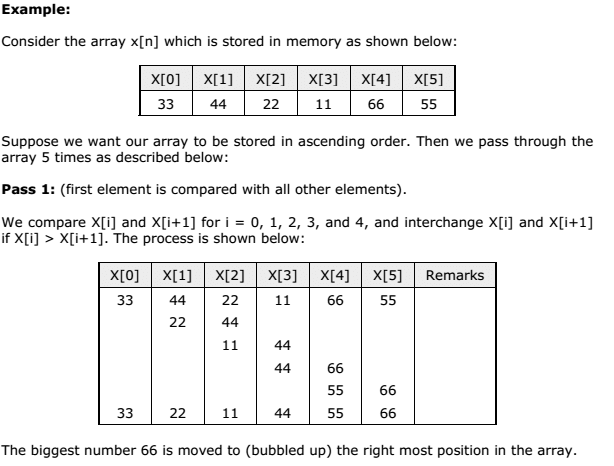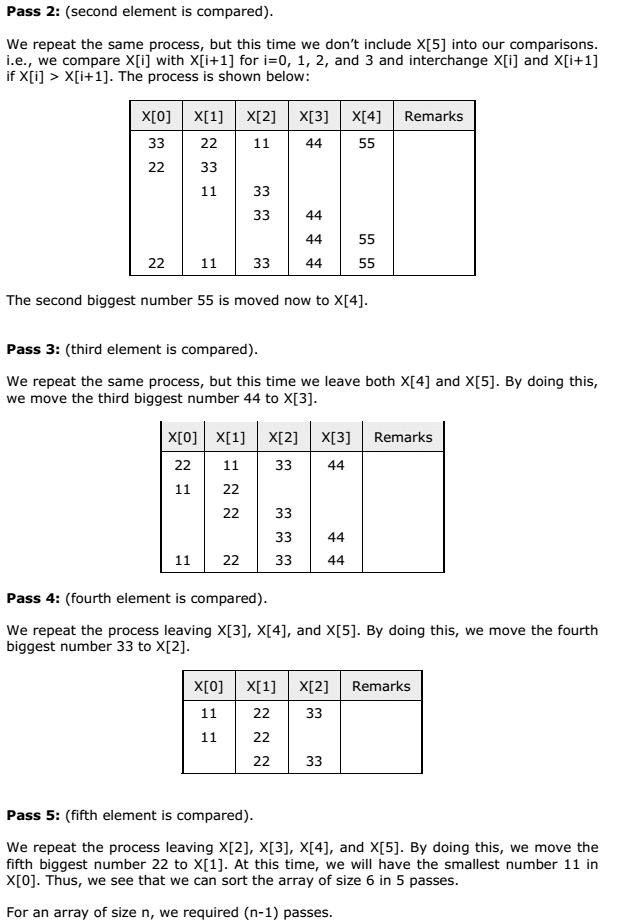The bubble sort is easy to understand and program. The basic idea of bubble sort is to pass through the file sequentially several times. In each pass, we compare each element in the file with its successor i.e., X[i] with X[i+1] and interchange two element when they are not in proper order. We will illustrate this sorting technique by taking a specific example. Bubble sort is also called as exchange sort.Time Complexity:

The bubble sort method of sorting an array of size n requires (n-1) passes and (n-1) comparisons on each pass. Thus the total number of comparisons is (n-1) * (n-1) = n^2 – 2n + 1, which is O(n^2 ). Therefore bubble sort is very inefficient when there are more elements to sorting.

You may be interested in:
Data Structures and Algorithms – MCQs.
Data Structures and Algorithms Online Tests